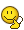# Lorentz transformation for an approaching observer

sukmeov
Homework Statement:
Let O and O' be observers both moving along the x-axis at constant velocity. Assume O and O' meet at the origin. Suppose also that O' moves towards O with relative velocity v. O reference frame has coordinates (x,t) and O' reference frame has coordinates (x',t') What is the Lorentz transformation for the two coordinate frames. ( I only care about the relationship between the time coordinates)
Relevant Equations:
Lorentz transform for positive velocity: t' = Lorentz factor * (1-v/c)t
I think this should be t'= Lorentz factor* (1+v/c)t, but that doesn't make sense to me.

sukmeov
Many thanks. So there is no sign change in the formula, even if v is negative? Also it's not a nickname it's my surname.

Many thanks. So there is no sign change in the formula, even if v is negative?

Also it's not a nickname it's my surname.I don't know what to say... goodbye PF it's been nice knowing you all•sukmeov
sukmeov
•I KNEW it!•